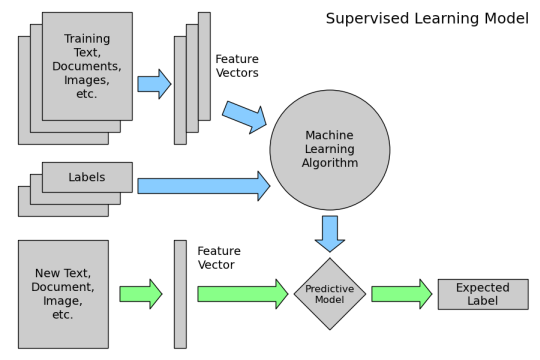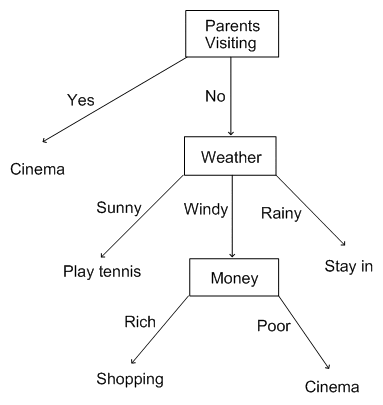# Machine Learning Algorithms: An OverviewMachine learning continues to advance at exponential rates. Algorithms that were once reserved for statisticians or mathematicians are now being democratized by the main players such as Microsoft with their Cognitive Services platform or IBM with their Watson ecosystem.

This is fantastic news for software development teams and other businesses that wish to leverage the power of machine learning into their daily operations without having to understand the nuts and bolts of the underlying algorithms that power these APIs.

Want the short version? Download our free “Common Machine Learning Algorithms” cheat sheet!

That said, it can be beneficial to understand some of the popular algorithms that are out there and what some of their applications are. That is exactly what we’ll cover in this blog post.

Before we jump into the algorithms, first, a few sentences on the different types of machine learning algorithms.

## Types of Machine Learning AlgorithmsSupervised learning:

Supervised learning can be explained as follows: use labeled training data to learn the mapping function from the input variables (X) to the output variable (Y).

Unsupervised learning:

Unsupervised learning problems possess only the input variables (X) but no corresponding output variables. It uses unlabeled training data to model the underlying structure of the data.

Reinforcement learning:

Reinforcement learning is a type of machine learning algorithm that allows the agent to decide the best next action based on its current state, by learning behaviors that will maximize the reward.

Source: KDNuggets

Now onto the algorithms!

## Naïve Bayes

Naïve Bayes is based on Bayesian Theorem and is a classification algorithm that uses probabilities to determine which category incoming data belongs to (based on its attributes).  The rule itself is written like this p(A|B) = p(B|A) p(A) / p(B) and is used as a means for arriving at predictions considering relevant evidence (it is also known as conditional probability or inverse probability.)

The theorem was discovered by an English Presbyterian and mathematician called Thomas Bayes and published posthumously in 1763.

The algorithm can be used in many use cases. For example, it can be used by email servers to detect spam email. It can even be used to perform sentiment analysis of social media data with reasonable accuracy, sometimes as much as 80% (depending on the quality of the training data).

Want the short version? Download our free “Common Machine Learning Algorithms” cheat sheet!

## Decision Trees

A decision tree is used for predictive modeling in machine learning and can be represented as a binary tree.  Because of this, decision trees are an optimal solution for making quick decisions with large datasets.  They can be deployed in many different use cases, and data that you supply to the tree generally doesn’t need any special pre-processing beforehand.

The decision tree assumes a tree-like structure with multiple nodes that contain Decisions and their possible Consequences.  Due to this structure, decision trees can be easy to understand for both technical and non-technical types.

In the image below, you can see a sample decision tree that contains decisions and the respective consequences.Decision trees have their own limitations and sometimes aren’t as accurate as other algorithms, additionally, a small change in training data can have a greater impact on predictions and therefore can make the decision tree brittle in terms of arriving at accurate predictions.

## Linear Regression

Linear regression belongs to the field of predictive modeling and its main purpose is to minimize errors or to make the most accurate prediction possible.  It was developed in the realm of statistics as a way of understanding the relationship between inputs and outputs and is both a machine learning algorithm and statistical algorithm.

It gets its name as the algorithm assumes a linear relationship between the input variables (X) and the single output value (Y), or to be more precise, that the value of (Y) can be determined from a linear combination of the input variables (X).  For example, you might want to predict someone’s weight (Y) from the input variable height (X).

Linear regression can often be called different things which can make your head spin!

For example, when you only have a single input variable (X), this may be referred to as simple linear regression, whereas if you have multiple input values, the world of statistics can refer to this as multiple linear regression.

## KNN (K-Nearest Neighbours)

The KNN algorithm is a classification algorithm with the main purpose of predicting which class new incoming data belongs to. It’s a non-parametric algorithm, meaning it doesn’t make any assumptions about the data.

KNN tries to make predictions for a new data point by searching through the entire training set for the K most similar instances (also referred to as feature similarity) and then summarizes the outputs for those K instances.  Loading and processing every feature can cause the KNN algorithm to consume considerable computing resources, however – something to be mindful of.

Things don’t often conform to the precise characteristics of predefined theoretical assumptions in real-world use cases. The KNN algorithm can be ideal for arriving at predictions in these types of situations where you have very little prior knowledge about the distribution data.

One scenario where KNN can be deployed is in the banking sector. For example, a bank can use KNN to determine if a new customer should be eligible for a credit card by collecting attributes of existing customers with creditworthy accounts and comparing it to the potential new account.

## Support Vector Machines (SVM)

Support Vector Machines (or SVMs) belong to the supervised learning family of machine learning algorithms.  They can be used to analyze data for regressions analysis and classification purposes.

The algorithm is supplied a set of training examples, each labeled as belonging to one or the other category. One example of this might be a list of positive sentences gets placed into one category, and a list of negative sentences get placed into the other.

It can be a useful algorithm to help you categorize text, images or even handwritten characters. In the biological and life sciences sector, the algorithm has been used to successfully classify proteins with up to 90% of the compounds being classified correctly!

Source: Machine Learning Mastery

It’s possibly one of the most powerful classifiers out there. You might want to try it out!

## Summary

In this blog post, we’ve looked at some of the popular machine learning algorithms that can be leveraged to help you classify data and make future predictions or recommendations.

We’ve touched on the pros and cons of these algorithms and explored some real-world scenarios where they can be used.  Maybe you can identify some uses cases in your business for these algorithms to help you find new insights in your data!

Want the short version? Download our free “Common Machine Learning Algorithms” cheat sheet!

Here at Growth Acceleration Partners, we have extensive expertise in many verticals.  Our nearshore business model can keep costs down whilst maintaining the same level of quality and professionalism you’d experience from a domestic team.